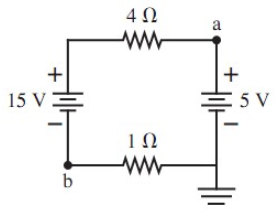# Problem: In the following figure, what is the value of the potential at points a and b?Express your answer using two significant figures.

###### FREE Expert Solution

(The symbol on the bottom right is a "ground". Since there's only one ground, current won't flow through it, but it acts as a reference point for zero potential.)

To analyze this circuit, use Ohm's Law:

$\overline{){\mathbit{V}}{\mathbf{=}}{\mathbit{i}}{\mathbit{R}}}$

And Kirchhoff's Loop Rule:

$\overline{){\mathbf{\sum }}{\mathbit{V}}{\mathbf{=}}{\mathbf{0}}}$

Assume current runs clockwise.

80% (360 ratings)###### Problem Details

In the following figure, what is the value of the potential at points a and b?